Pure and Applied Mathematics Journal
Volume 4, Issue 5, October 2015, Pages: 219-224

Solving Fourth Order Parabolic PDE with Variable Coefficients Using Aboodh Transform Homotopy Perturbation Method

Khalid Suliman Aboodh

Department of Mathematics, Faculty of Science & technology, Omdurman Islamic University, Khartoum, Sudan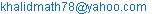Khalid Suliman Aboodh. Solving Fourth Order Parabolic PDE with Variable Coefficients Using Aboodh Transform Homotopy Perturbation Method. Pure and Applied Mathematics Journal. Vol. 4, No. 5, 2015, pp. 219-224. doi: 10.11648/j.pamj.20150405.13

Abstract: Here, a new method called Aboodh transform homotopy perturbation method (ATHPM) is used to solve one dimensional fourth order parabolic linear partial differential equations with variable coefficients. The proposed method is a combination of the new integral transform "Aboodh transform" and the homotopy perturbation method. Some cases of one dimensional fourth order parabolic linear partial differential equations are solved to illustrate ability and reliability of mixture of Aboodh transform and homotopy perturbation method. We have compared the obtained analytical solution with the available Aboodh decomposition solution and homotopy perturbation method solution which is found to be exactly same. The results obtained reveal that the combination of Aboodh transform and homotopy perturbation method is quite capable, practically well appropriate for use in such problems.

Keywords: Aboodh Transform, Homotopy Perturbation Method, Linear Partial Differential Equation

Contents

1. Introduction

Many problems of physical interest are described by linear partial differential equations with initial and boundary conditions. One of them is fourth order parabolic partial differential equations with variable coefficients; these equations arise in the transverse vibration problems . In recent years, many research workers have paid attention to find the solution of these equations by using various methods. Among these are the variational iteration method [Biazar and Ghazvini (2007)], Adomian decomposition method [Wazwaz (2001) and Biazar et al (2007)], homotopy perturbation method [Mehdi Dehghan and Jalil Manafian (2008)], homotopy analysis method [Najeeb Alam Khan, Asmat Ara, Muhammad Afzal and Azam Khan (2010)] and Laplace decomposition algorithm [Majid Khan, Muhammad Asif Gondal and Yasir Khan (2011)]

Prem Kiran G. Bhadane, and V. H. Pradhan (2013)]. In this paper we use coupling of new integral transform "Aboodh transform" and homotopy perturbation method. This method is a useful technique for solving linear and nonlinear differential equations. The main aim of this paper is to consider the effectiveness of the Aboodh transform homotopy perturbation method in solving higher order linear partial differential equations with variable coefficients. This method provides the solution in a rapid convergent series which leads the solution in a closed form.

2. Aboodh Transform Homotopy Perturbation Method [1, 2, 3, 4]

Let us consider the a one dimensional linear nonhomogeneous fourth order parabolic partial differential equation with variable coefficients as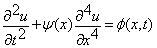(1)

where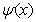is a variable coefficient, with initial conditions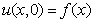and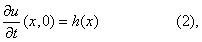and boundary conditions as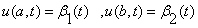,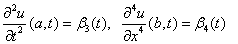(3)

Making use the Aboodh transform on both sides of

Eq. (1), we obtain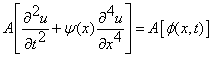(4)

and, Aboodh transform of partial derivative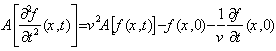With the aid of this property, Eq. (3) as redas(5)

By using of initial conditions in Eq. (5), we haveor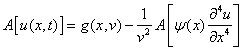(6)

Where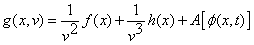Applying Aboodh inverse on both sides of Eq. (6), admits to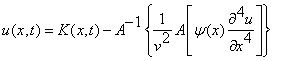(7)

Where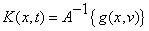, represents the term

Arising from the source term and the prescribed initial conditions.

By apply the homotopy perturbation method.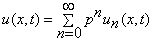(8)

By substituting Eq. (8) into Eq. (7), we get(9)

This is the coupling of the Aboodh transform and the homotopy perturbation method. collectiy the coefficient of like powers of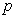, we haveAnd so on

In general recursive relation is given by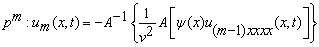Then the solution can be expressed as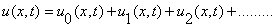(10)

3. Application

In this section, to demonstrate the effectiveness of the proposal method we consider homogeneous and non homogeneous one dimensional fourth order linear partial differential equations with initial and boundary conditions.

Example 1.

Let us first consider fourth order homogeneous partial differential equation as [1,5]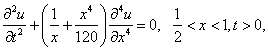(11)

With initial conditions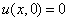and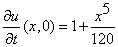(12)

and boundary conditions(13)

Applying Aboodh transform to Eq. (11), admits to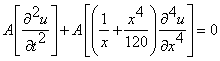Using initial conditions from Eq. (12), we haveBy used Aboodh inverse on both sides of above Eq. (15),To solve Eq.(12) using  homotopy perturbation method,

we assume that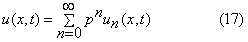inserty Eq ( 17) of u(x, t) into Eq. (16), we getCollecting the coefficient of like powers of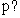in Eq. (18) we haveand so on in the same manner the rest of the components of iteration formula can be obtained and thus solution can be written in closed form aswhich is an exact solution of Eq. (11) and can be verified through substitution

Example 2.

A second instructive model is the fourth order homogeneous partial differential equation as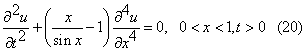with the initial conditions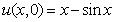and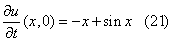and boundary conditionsApplying Aboodh transform to Eq. (20), we get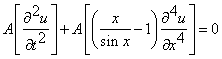Using initial conditions from Eq. (20), we getNow taking Aboodh inverse on both sides of above

Eq. (24), we haveNow, we apply the homotopy perturbation method.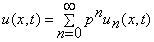Putting this value of u(x, t) into Eq. (25), we getcollecty the coefficient of like powers of p , in Eq. (26) the following approximations are obtainedand so on in the same manner the rest of the components of iteration formula can be obtained and thus solution can be written in closed form asWhich is an exact solution of Eq. (20) and can be

Verified  through substitution

Example 3.

in this case we consider the  fourth order nonhomogeneous partial differential equation as [1,5]with the following initial conditions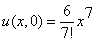and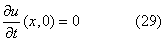and boundary conditionsApplying Aboodh transform to Eq. (28), we getUsing initial conditions from Eq. (29), we getnow taking Aboodh inverse on both sides of above Eq. (31),we haveNow, we apply the homotopy perturbation method.Putting this value of u(x, t) into Eq. (32), we getHere, we assume that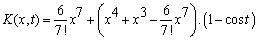Can be divided into the sum of two parts namely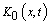and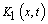therefore we getUnder this assumption, we propose a slight variation only in the components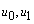. The variation we propose is that only the partbe assigned to the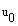where as the remaining part, be combined with the other terms to define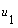.In view of these, we formulate the modified recursive algorithm as followsAnd so on in the same manner the rest of the components of iteration formula can be obtained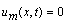for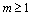Thus solution can be written in closed form asWhich is an exact solution of Eq. (28) and can be verified through substitution

4. Conclusion

The aim of this paper is to show the applicability of the mixture of new integral transform "Aboodh transform" with the homotopy perturbation method to solve one dimensional fourth order homogeneous and nonhomogeneous linear partial differential equations with variable coefficients. This combination of two methods successfully worked to give very reliable and exact solutions to the equation. This proposed method provides an analytical approximation in a rapidly convergent sequence with in exclusive manner computed terms. Its rapid convergence shows that the method is trustworthy and introduces a significant improvement in solving linear partial differential equations over existing methods.

Finally it's worthwhile to mention that the proposed method can be applied to other linear and nonlinear partial differential equations arising in mathematical physics. This aim task in future.

References

1. Majid Khan, Muhammad Asif Gondal and Yasir Khan, An Efficient Laplace Decomposition algorithm for Fourth order Parabolic Partial Differential Equations with variable coefficients, World Applied Sciences Journal 13 (12), 2011, pp2463-2466.
2. Tarig M. Elzaki and Eman M. A. Hilal, Homotopy Perturbation and ELzaki Transform for solving Nonlinear Partial Differential equations, Mathematical Theory and Modeling, 2(3),2012, pp33-42.
3. Khalid Suliman Aboodh, Application of New Transform "Aboodh Transform" to Partial Differential Equations ''Global Journal of Pure and Applied Mathematics ISSN 0973-1768 Volume 10, Number 2 (2014), pp. 249-254.
4. Hradyesh Kumar Mishra and Atulya K Nagar, He-Laplace Method for Linear and Nonlinear Partial Differential Equations, Journal of Applied Mathematics, Vol.2012,Article ID 180315, 16 pages.
5. Mehdi Dehghan and Jalil Manafian, The Solution of the Variable Coefficients Fourth-Order Parabolic Partial Differential Equations by the Homotopy Perturbation Method,Verlag der Zeitschrift fur Naturforschung, Tu¨bingen, 64a, 2009, pp420-430.
6. M. Hussain and Majid Khan, Modified Laplace Decomposition Method, Journal of Applied Mathematical Sciences, 4(36), 2010, pp 1769-1783.
7. Najeeb Alam Khan, Asmat Ara, Muhammad Afzal and Azam Khan, Analytical Aspect of Fourth order Parabolic Partial differential Equations with variable coefficients, Mathematical and Computational Applications, 15(3), 2010, pp. 481-489.
8. Khalid Suliman Aboodh, The New Integral Transform ''AboodhTransform''GlobalJournalofPure and Applied Mathematics ISSN0973-1768 Volume 9, Number 1 (2013), pp. 35-43.
9. Tarig M. Elzaki and Salih M. Elzaki, Applications of New Transform "ELzaki Transform" to Partial Differential Equations,Global Journal of Pure and Applied Mathematics, (7)1,2011,pp65-70.
10. He J. Homotopy-perturbation method for solving boundary value problem, Phys Lett A, 350 (2006), 87-88.

 Contents 1. 2. 3. 4.
Article ToolsAbstractPDF(185K)